## How do I put variables from a maple worksheet into...

I'm not sure if this can even be done, but if it's possible, how do I do it:

I have a python script called "Blue.py", the path should simply be "Blue.py", since my worksheet and the python script are saved in the same place.

I want to somehow import two arrays and an equation from maple to said python script, use them in a procedure there, and then import the output of said procedure, a 2d array, backwards to maple.

I don't really care what format the equation and the arrays take in python (the current version assumes types "np.array" for the arrays and type procedure for the equation, but I can always adjust it), but the type of the output of Blue.py should be of the form 2d Array (for example [[0,1],[8,-2],[3,3]] ).

Is it possible to shuffle input between maple worksheets and python scripts like that, and if so, how?

## What is the proof that Neile's semi-cubical parabo...

The uploaded worksheet is a failed attempt at this proof.

Please either refer me to or show me a valid proof.

*** I have tried to upload my worksheet using the green arrow but the insert link operation of the upload failed ***

ccError occurred during PDF generation. Please refresh the page and try again

## How do I evaluate double integral with sybolic lim...

int(int(x^(alpha + 1)*y^(beta + 1)*exp(-(x^2 + y^2)/(4*Pi))/sqrt(x^2 + y^2), x = -R .. R), y = -R..R) assuming x>0, y>0, R>0;

I am trying to evaluate the above symbolic integral but Maple returns the same input in Symbolic form without evaluating it at all. Is there something wrong with my approach?## How do I implement the convolution integral of 2 c...

Hi there!

I'm attempting to develop a custom library in MapleSim (a Modelica solver engine) that can use convolution integrals to model the hydrodynamic behaviour of floating bodies. The convolution integral is mathematically represented as follows: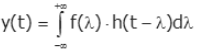And the equation I'm particularly interested in solving is as follows:I'm trying to solve this convolution integral in Modelica using a model that can be imported to MapleSim. So far, I've had no luck in implementing this convolution for continuous functions symbolically. I have used a numerical approach on 2 arrays using the following approach in Modelica:

``````// Modelica function
function convIntegral
input Real simTime;  // Simulation time
input Real f[:];     // Kernel function array
input Real g[:];     // Second function array

output Real h[(2*simTime) - 1];    // Output the convolution integral in the form of an array

// Define the algorithm to numerically compute the convolution integral of 2 arrays
algorithm
// Initialize the output array with zeroes
for i in 1:((2*simTime) - 1) loop
h[i] := 0;
end for;

// Iterate over the simulation time
// Recursively increment the convolution array with the pointwise product of the 2 functions
for i in 1:simTime loop
for j in 1:simTime loop
h[i+j-1] := (f[i] * g[j]) + h[i+j-1];
end for;
end for;
end convIntegral;
// End of function to compute the convolution integral``````

This works perfectly for discrete samples and I have verified it with output from Matlab's inbuilt function

``conv(A,B)  % For 2 arrays A and B``

However, I would like to implement this on 2 continuous functions and this numerical approach does not work since MapleSim does not support conversion between discrete and continuous signals.

I understand that convolution is essentially an operation between two functions, where we time-flip one of the functions (kernel) and then slide it across the other function while measuring the bounded area and outputting that as the result of the convolution. I include this image from Wikipedia that sums up convolution: (Not including links as they mark questions as spam)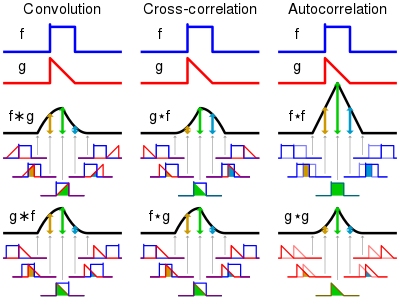I've tried implementing this in Modelica using the following code:

``````// Model to perform convolution on 2 continuous functions
model ConvolutionalIntegral

extends Modelica.Blocks.Icons.Block;

// Define model variables
Real kernelFunction = (e ^ (-0.5 * time)) * cos(0.5 * time);  // I've taken an example of a function I might use
Real kernelFunctionFlipped = (e ^ (-0.5 * (T_sim - time))) * cos(0.5 * (T_sim - time));  // I've flipped the kernel function about the vertical axis by converting the (time) variable to (T_sim - time) where (T_sim) is a variable storing the simulation duration
Real secondFunction;  // The other function for the convolution
Real convolutionIntegralOutput;  // Function to store the output

equation
// Convolution implementation
der(convolutionIntegralOutput) = kernelFunctionFlipped * secondFunction;

// Final equation to solve
der(secondFunction) + convolutionIntegralOutput = 0;
// An example of a differential equation I'd like to solve involving the convolution integral

end ConvolutionIntegral;``````

I had hoped that this would yield the output of the convolution since I'm essentially multiplying the time-flipped kernel and the other function and then integrating them over time. However, the output does not provide the expected result and it appears that Modelica interprets my code to mean that I'm integrating the pointwise product of these 2 functions over time instead of sliding the kernel over the other function.

I'd appreciate it if you could take a look at my code and my approach to solving the convolution integral symbolically, and point out where I'm making a mistake and what a possible fix might be.

Thank you!

## why calling deep call on object makes it no longer...

This is another bizzar behaviour of objects. I created a basic object of type person.  Noticed that after issuing the call copy(object,deep) and not doing anything at all with the result. Just made the call only, then I am not longer able to create new objects of this class. I had to restart the session to be able to create new objects again.

Why?  This seems like something went messy with memory layout. I put a print message in the constructor, and see that this message no longer show up  after the call copy(object,deep) even though this call was not used for any purpose as you see, but to only to see its effect on the session.

Any explanation why one can no longer create new objects after doing this?  Here is the workseet

 > interface(version)> restart;
 > person:=module()     option object;     export name::string:="";       export ModuleCopy::static:=proc(_self,proto, name::string, \$)       print("Enter constructor of person");       _self:-name := name;     end proc; end module;> p1:=Object(person,"me"); p1:-name; do_not_care := copy(p1,deep);> p2:=Object(person,"me");  #WHy constructor no long called? p2:-name;   #this no longer work.> p3:=Object(person,"me");  #WHy constructor no long called? p3:-name;   #this no longer work.> restart;  #had to call restart to fix things.
 > person:=module()     option object;     export name::string:="";       export ModuleCopy::static:=proc(_self,proto, name::string, \$)       print("Enter constructor of person");       _self:-name := name;     end proc; end module;> p4:=Object(person,"me");  #now it works again p4:-name;>

## How to remove the mosaic of vertices?...

I  generated some graphs via maple and would like to put them in my paper. So I am going to convert the following worksheet to pdf.

```with(GraphTheory):
Graphs:=[NonIsomorphicGraphs(6,8,output=graphs,outputform = graph)]:
num_g:=nops(Graphs):
num:=ceil((num_g)/5.):
M1:=Matrix (num,5,(i,j)->`if`((i-1)*5+j<=num_g, DrawGraph(Graphs[(i-1)*5+j],size=[250,250] ,overrideoptions ,showlabels=false,style=planar, stylesheet =  [
vertexcolor     = orange
,vertexfontcolor = black
,vertexborder    = false
,edgethickness   = 0.6
,edgecolor       = MidnightBlue
,vertexshape     =  "circle"
,vertexfont      = [Arial, 4],
vertexthickness=5], caption = cat(H__,5*(i-1)+j),captionfont=["ROMAN",7]),plot(x = 0 .. 1, axes = none))):
DocumentTools:-Tabulate (M1[1..5,.. ],widthmode=percentage ,width=80 , exterior =all) :
```

But there was a problem with the exported pdf. There was some mosaic stuff at the vertices of all those graphs. It was strange. (I want to reduce the size of vertices of the graphs in order not to look crowded.)Only when I insert the option vertexpadding and set a large enough size  of vertex (for this example, we set vertexpadding=7),  it won't go wrong. However, in fact, we often need make vertice‘s size smaller , especially when there are more vertices.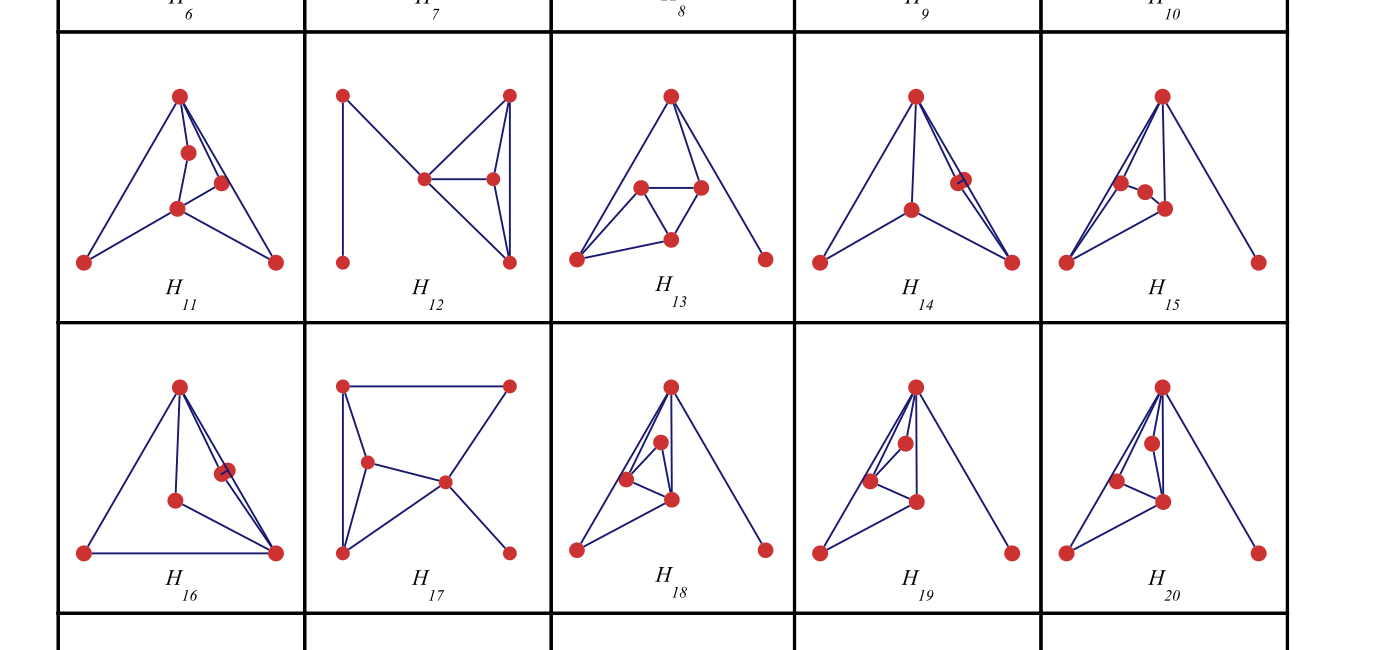## passing the object itself to one of its method ca...

As I was trying things with Maple OOP, I noticed strange behavior which I do not understand. I'll explain the problem in words first then given an example.

I have Person class. Then made one instance of it. In this class, there is one method which is defined to take in an object of same type as the class itself.

I found when I pass the object itself to its own method, it gives an error that the method expects a second argument of type person which is missing. But it is not missing.

Then when creating a second object of same type, and passing the second object, it worked!

Why?  Here is MWE. Attached worksheet.  I would have expected same behavior in both cases. EIther both work, or both do not work. As as long as the object being passed is the correct type (person).

It seems in the first case, Maple noticed the object being passed to the method happened to be same object where this method is, and it did not pass it. Hence the missing second argument.  Is this a documented behaviour somewhere? (I made sure to make all method static though, so same code is used by all instances of the class. This is strange error message).

 > interface(version)> restart;
 > person:=module()     option object;     export name::string:="";       #--- constructor---     export ModuleCopy::static:=proc(_self,proto, name::string, \$)       _self:-name := name;     end proc;     export process::static:=proc(_self, the_input::person ,\$)        print("in person::process. name = ", the_input:-name);     end proc; end module;> p:=Object(person,"me");> p:-process(p);  #why this fail

Error, invalid input: process uses a 2nd argument, the_input (of type person), which is missing

 > o:=Object(person,"new_name"); #make new object and try again> p:-process(o); #why this OK?>

Update

Ok, I am all set. I found a good way to do this. i.e. pass the object to one of its method. One has to make a copy of the object first and pass the copy. Can't use the same object. This is how I do it now.

```restart;
person:=module()
option object;
export name::string:="";

[
print("Enter 2 args constructor of person");
#do nothing
end proc,

print("Enter 3 args constructor of person");
_self:-name := name;
end proc
]);

export process::static:=proc(_self, the_input::person,\$)
print("the_input:-name = ",the_input:-name);
end proc;

end module;

p:=Object(person,"me");
#p:-process(p);  #instead of this do the following
o:=Object(p);
p:-process(o)
```

Which works and gives

```              "Enter 3 args constructor of person"
p := Object<<2370600065568>>
"Enter 2 args constructor of person"
o := Object<<2370600048288>>
"the_input:-name = ", "me"

```

So I am now able to pass the object (or rather copy of it) as explicit argument to other methods and other modules.  I am not sure why it does not work when using the object itself and had to make a copy.

## How to integrate the given function...

How to integrate the below function from 0 to eta.

A := P(eta)+S(H-2*`cos&theta;`(eta+1)*F)+`cos&theta;`*(2*F(eta)-2*H*F) = S(eta+1)*`sin&theta;`*F(theta)-`sin&theta;`(F*H(theta)-H*F(theta)+F(theta, eta))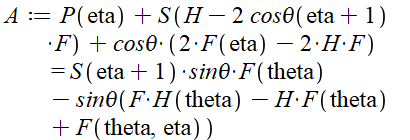How does one undo an overload?

## How to solve ODE?...:RLC circuit I want to solve.(1)(2)(3)(4)(5)(6)(7)

How to solve this by Maple? The answer I want to solve is the plot shown above.

## Simpson and trapezoidal rule for definite integral...

Hi experts, I know built in command to solve definite integral by using simpson and trapezoidal rule but i need to show step which is given in the example to satisfy his students of grade 12. Please help me to solve this example## Why does my Maple animation only work for 120 fram...

I have an animation in Maple that only works if I use the option frames=120, or anything >120. Anything less and it gives the error "Error, (in plots/animate) points cannot be converted to floating-point values". I don't understand why this restriction is happening, any advice is appreciated.

## Is there a better way to impletment a transparent...

transparent_background_in_plots.mw

Is there a better way to impletment a transparent background when genereating plots?

Here I generate a plot with a yellow background.

plot(sin(x), x = 0 .. 4*Pi, background = yellow);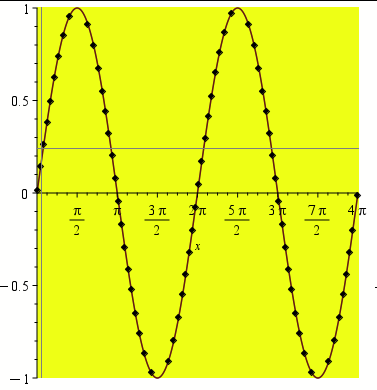I want the background to be completely transparent instead of yellow.

plot(sin(x), x = 0 .. 4*Pi, background = none);

Error, (in plot) Unable to find image file, none

none is not an accepted color, so an error is returned. Is there such a color as "clear" that is fully transparent? Is there a better way to impletment a transparent background when genereating plots?

## The NormalSeries of maple is different with textb...

As we know, when every Gi is normal in G, then the series is called a normal series:

`cs := CompositionSeries(DihedralGroup(8))`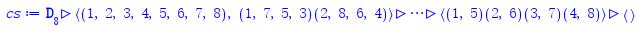We can draw it:

`DrawSubgroupLattice(DihedralGroup(8), labels = ids, highlight = cs)`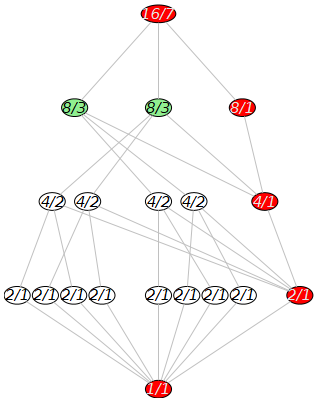And all Gi is normal in G:

`IsNormal~(cs, DihedralGroup(8))`

[true, true, true, true, true]

But why `type(cs, 'NormalSeries')` will get `false`.. It is a bug or do I have a misunderstanding?

## How to call parent class constructor from sub clas...

I am still struggling understanding Maple Object  inheritance due to lack of good examples and sparse documenation.

This is what I am trying to do. I have base class Person, and then make Employee class which extends Person class and adds new field.

The constructor to the Employee class then needs to call the constructor to the Person class (its base class) in order to initialize it. The base class constuctor takes care of initialization the fields in the base class.

I do not know how to do this. Actually it is not clear to me how the proto argument gets into play in all of this. I could not find one example that explains how to use proto with inheritance.

I am trying to implement this python example. I will show the code for the Python, and my Maple translation. The part I do not know how to do, it how to call the constructor for the base class from the sub class.  If I do not do this, then the fields of the base class will remain unitilized.

Python example is from https://www.geeksforgeeks.org/python-access-parent-class-attribute/

```# parent class
class Person( object ):

# __init__ is known as the constructor
def __init__(self, name, idnumber):
self.name = name
self.idnumber = idnumber

def display(self):
print(self.name)
print(self.idnumber)

# child class
class Employee( Person ):
def __init__(self, name, idnumber, salary):
self.salary = salary

# invoking the constructor of the parent class
#----->>>>> HOW TO DO THIS IN MAPLE?
Person.__init__(self, name, idnumber)

def show(self):
print(self.salary)

# creation of an object
# variable or an instance
a = Employee('Rahul', 886012, 30000000)

# calling a function of the
# class Person using Employee's
# class instance
a.display()
a.show() ```

Here is the Maple code

```restart;
person:=module()
option object;
#I made these exported instead of local for ease of printing from outside while
#debugging , that is all.. These should otherwise be local

export idnumber::integer:=0;
export name::string:="";

export ModuleCopy::static:= proc( _self, proto, name::string, idnumber::integer, \$)
_self:-idnumber := idnumber;
_self:-name := name;
end proc;

end module;
#---- extend the above class

employee:=module()
option object(person);
export salary::integer:=0;

ModuleCopy::static:= proc( _self, proto, name::string, idnumber::integer, salary::integer , \$)
_self:-salary := salary;
# invoking the constructor of the parent class
# How to do that in Maple?

#I could always manually do this. But I do not want to duplicate the code
#done by the base class constructor.
#_self:-name := name;
#_self:-idnumber := idnumber;

end proc;

end module;

```

How does one do the Python example in Maple?

Someone should write a book on programming using OOP in Maple. I'll buy a copy in advance.

Maple 2022.1

﻿